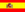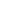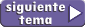home | más de estática | otros temas de Física | lecciones del maestro Ciruela | tonterías | @NO ME SALEN
APUNTES TEÓRICOS Y EJERCICIOS RESUELTOS DE FÍSICA DEL CBC
Center of MassSpeed of the Center of Mass

One of the most interesting properties of the center of mass appears when taking into account a body-system moving in different directions, as a group of stars in a galaxy, remnants of an explosion, etc.

Before I told you that the center of mass can be found by calculating a “weighted average” between all the positions, xi, that occupies each of the mass fragments, mi, that integrate a body or alternatively a mass system.

 xG = Σ mi xi M

Where M is the total mass of the sistem. When the mass distribution is found on a plane or in a volume the process to find the 2 or 3 coordinates of the center (x, y, z) of mass is repeated.

On this section, as to abbreviate I’m going to call ri to the position of each fragment independently of how is the general distribution. So:

 r (with an arrow above) is the position vector, and is far more versatile that the coordinate. But I can’t seem to find the arrow for the r in HTML. Que pena.
 rG = Σ mi ri M

Let’s do a simple algebraic transformation that’ll reveal a surprising property. First, we make M (the total mass) multiply on the left:

M rG = Σ mi ri

Now let’s suppose everything is in movement: the positions of the fragments change, and of course, so do the ones of the center of mass. This could be interpreted – kind of rudely- like this:

M ΔrG = Σ mi Δri

Now, if we divide each side by a period of time in which the displacement occurs, we’ll have:

 M ΔrG = Σ mi Δri Δt Δt

 Do me a favour: Always remember the arrow above v and r (both are vectors)

Or, what’s the same:

M vG = Σ mi vi

Surprise:

 pG = Σ pi

 Same goes for p (quantity of movement)

This tells us that the quantity of movement of the center of mass equals the sum of all the movement of each fragment of mass that makes the body or system (but don’t forget: we are dwelling with a vector sum.

From this relation we deduce surprising relations. Take: If a system of particles doesn’t receive external forces, then the speed of the center of mass will be null or either remain in movement at constant speed.

Take this: If all the particles integrating a system receive exactly the same force, whatever they

do, the center of mass will behave as if all the mass was reunited in that spot and there, just there, was receiving that external force.

If you want to know about the speed of the center of mass, then we only have to divide by the total mass on the right.

 vG = Σ mi vi / M

If you were against a two or more dimentions problem, then you’ll have to solve the equation for each of the speed components. Take:

vGx = Σ mi vix / M

vGy = Σ mi viy / M

Then you recompose the speed since its components.

Important Gossip:
• The correct deduction of these relations is done with the help of mathematicalanalysis. And in the same way it’s proven that the acceleration of the center of mass equals the sum of the “weighted accelerations” of the total fragments.
• Cyclists win races by pulling their bodies backwards at the last second. At that brief moment just before crossing the finishing line the bike suffers a strong forward acceleration and thus the cyclist wins the race. However the center of mass of the two bodies (cyclist-bike) didn’t vary in speed.
• A diver springs from the springboard and spins while falling, doing curlicues and 248 other pirouettes. The speed and acceleration of each of his parts: arms, hands, head, waist, legs, ears, teeth and facial hair, remain chaotic and is impossible to keep track. However the center of mass of the diver describes a clean and pure trajectory: a perfect parable.Trick Questions:
• How can an explosion be physically described?
• How would you put the property of this lesson as a conservation law?Translated by Román Dumas. Some Rights Reserved. Not allowed to be copied without naming either the author or this source material. Last Updated nov-15. Buenos Aires, Argentina.